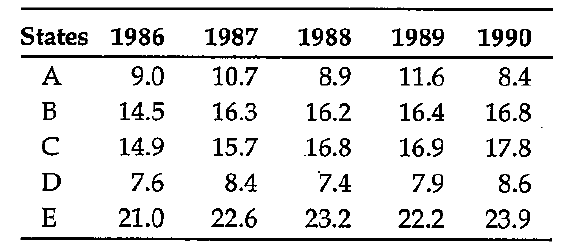# Data Interpretation - Tabular Data

>>>>>>>>Tabular Data

Instructions:

Study the data given in the following table carefully and answer the questions given below it: Wheat Production (in lakh tonnes)• A

A• B

B• C

C• D

D• Option : A
• Explanation : Total production of all the five states in 1988 = 72.5

1/8th of 72.5 = 9 (approx.)

State A contributes close to 1/8th of the total production of all the five states.

Instructions:

Study the data given in the following table carefully and answer the questions given below it: Wheat Production (in lakh tonnes)• A

1986• B

1987• C

1988• D

1989Instructions:

Study the data given in the following table carefully and answer the questions given below it: Wheat Production (in lakh tonnes)• A

A• B

B• C

C• D

D• Option : A
• Explanation : Percentage increase in production of State A in 1989 over that in 1986 = (2.6/9) * 100 = 28.89%

Similar figures for the other states are 13.10, 13.42, 3.95, 5.71 respectively

Instructions:

Study the data given in the following table carefully and answer the questions given below it: Wheat Production (in lakh tonnes)• A

1986• B

1987• C

1988• D

1990• Option : D
• Explanation :

% Increase of production in 1987 over that in 1986 = (1.6/21) * 100 = 7.62. Similar figures in 1988 over that in 1987 = 2.65 and in 1990 over that in 1989 is 7.66

Instructions:

Study the data given in the following table carefully and answer the questions given below it: Wheat Production (in lakh tonnes)• A

A• B

B• C

C• D

DRelated Quiz.
Tabular Data